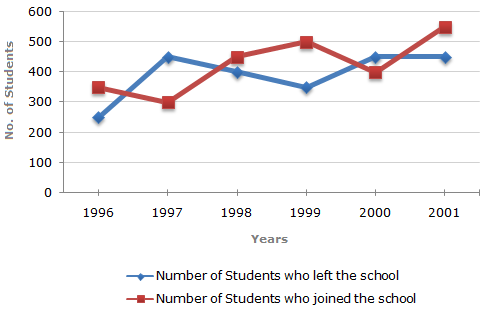# Data Interpretation - Line Charts

Exercise : Line Charts - Line Chart 9
Directions to Solve

Study the following line graph which gives the number of students who joined and left the school in the beginning of year for six years, from 1996 to 2001.

Initial Strength of school in 1995 = 3000.1.
The number of students studying in the school during 1999 was?
2950
3000
3100
3150
Explanation:

As calculated above, the number of students studying in the school during 1999 = 3150.

2.
For which year, the percentage rise/fall in the number of students who left the school compared to the previous year is maximum?
1997
1998
1999
2000
Explanation:

The percentage rise/fall in the number of students who left the school (compared to the previous year) during various years are:

 For 1997 =(450 - 250) x 100% = 80% (rise). 250

 For 1998 =(450 - 400) x 100% = 11.11% (fall). 450

 For 1999 =(400 - 350) x 100% = 12.5% (fall). 400

 For 2000 =(450 - 350) x 100% = 28.57% (rise). 350

 For 2001 =(450 - 450) x 100% = 0%. 450

Clearly, the maximum percentage rise/fall is for 1997.

3.
The strength of school incresed/decreased from 1997 to 1998 by approximately what percent?
1.2%
1.7%
2.1%
2.4%
Explanation:

Important data noted from the given graph:

In 1996 : Number of students left = 250 and number of students joined = 350.

In 1997 : Number of students left = 450 and number of students joined = 300.

In 1998 : Number of students left = 400 and number of students joined = 450.

In 1999 : Number of students left = 350 and number of students joined = 500.

In 2000 : Number of students left = 450 and number of students joined = 400.

In 2001 : Number of students left = 450 and number of students joined = 550.

Therefore, the numbers of students studying in the school (i.e., strength of the school) in various years:

In 1995 = 3000 (given).

In 1996 = 3000 - 250 + 350 = 3100.

In 1997 = 3100 - 450 + 300 = 2950.

In 1998 = 2950 - 400 + 450 = 3000.

In 1999 = 3000 - 350 + 500 = 3150.

In 2000 = 3150 - 450 + 400 = 3100.

In 2001 = 3100 - 450 + 550 = 3200.

Percentage increase in the strength of the school from 1997 to 1998

 =(3000 - 2950) x 100% = 1.69%1.7%. 2950

4.
The number of students studying in the school in 1998 was what percent of the number of students studying in the school in 2001?
92.13%
93.75%
96.88%
97.25%
Explanation:

 Required percentage =3000 x 100% = 93.75% 3200

5.
The ratio of the least number of students who joined the school to the maximum number of students who left the school in any of the years during the given period is?
7:9
4:5
3:4
2:3